﻿ Charting with Multiple Plot Areas# Charting with Multiple Plot Areas

Stacking plot areas within a chart can be a useful way to better analyze the data points. Rather than having many data series overlapped you can increase readability by moving some series to an adjacent plot area. This can all be achieved using the advanced capabilities of the ComponentOne Chart for XAML.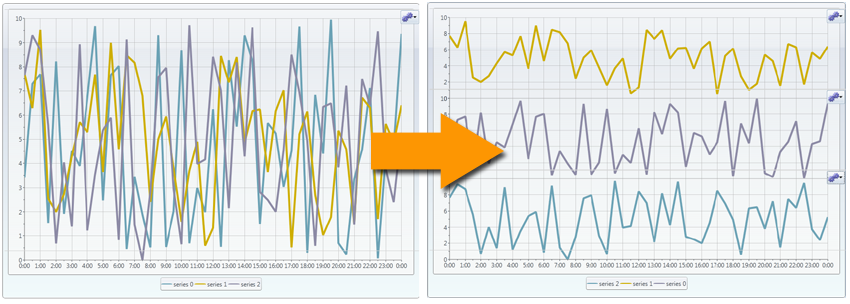In this post I will first introduce the plot area feature. You’ll learn how they work and how you can customize them in XAML and in code. And then I’ll show you an advanced sample that demonstrates run-time plot area manipulation. In this advanced sample the user has the power to create as many plot areas as he/she wants and can drag/drop data series among each plot. It’s pretty amazing!

# Plot Areas Introduction

Showing more than one chart control side-by-side is nothing more than trivial. But when you have the ability to stack multiple plot areas within the same chart you are able to share common regions such as the axes and legends. With C1Chart, plot areas can be created by simply creating a new auxiliary Axis and assigning it a new PlotArea. So to create a new plot area you follow these steps:

2. Increment the axis’ PlotAreaIndex value (based the number of current plot areas)
``````
<c1:C1Chart ChartType="Column" Name="c1Chart1">
<c1:C1Chart.Data>
<c1:ChartData ItemNames="P1 P2 P3 P4 P5">
<c1:DataSeries Label="s1" Values="20, 22, 19, 24, 25" />
<c1:DataSeries Label="s2" Values="8, 12, 10, 12, 15" />
</c1:ChartData>
</c1:C1Chart.Data>
<c1:C1Chart.View>
<c1:ChartView>
<!-- Aux axis -->
<c1:Axis x:Name="y2" Title="y2"
AxisType="Y" PlotAreaIndex="1" />
</c1:ChartView>
</c1:C1Chart.View>
<c1:C1ChartLegend />
</c1:C1Chart>

``````

Notice in line #11 we declare our auxiliary “y2” axis. The chart now looks like this: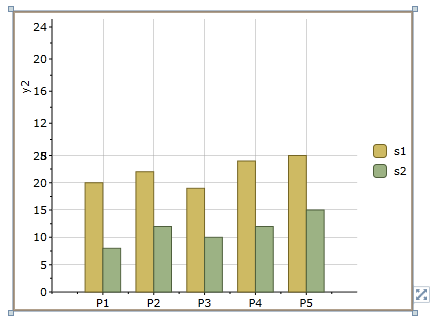A data series is rendered in the plot in which its axis lies. To move a data series to the new plot, you simply set the AxisX or AxisY property on that series to the auxiliary axis. Here is the updated XAML:

``````
<c1:C1Chart ChartType="Column" Name="c1Chart1">
<c1:C1Chart.Data>
<c1:ChartData ItemNames="P1 P2 P3 P4 P5">
<c1:DataSeries Label="s1" Values="20, 22, 19, 24, 25" />
<c1:DataSeries Label="s2" Values="8, 12, 10, 12, 15" AxisY="y2" />
</c1:ChartData>
</c1:C1Chart.Data>
<c1:C1Chart.View>
<c1:ChartView>
<!-- Aux axis -->
<c1:Axis x:Name="y2" Title="y2"
AxisType="Y" PlotAreaIndex="1" />
</c1:ChartView>
</c1:C1Chart.View>
<c1:C1ChartLegend />
</c1:C1Chart>

``````

Notice in line #5 we set that DataSeries’ AxisY property to our y2 axis. This effectively moves the series to the plot area in which that axis lies.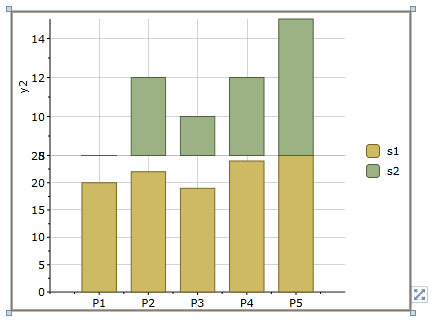Not only can we stack plot areas vertically but we can stack horizontally too. This is controlled by whether you are adding X or Y axes. To stack horizontally we would add an auxiliary X axis. Here is an example:

``````
<c1:C1Chart ChartType="Area" Name="c1Chart1">
<c1:C1Chart.Data>
<c1:ChartData>
<c1:DataSeries Label="s1" Values="20, 22, 19, 24, 25" />
<c1:DataSeries Label="s2" Values="8, 12, 10, 12, 15" AxisX="x2" />
</c1:ChartData>
</c1:C1Chart.Data>
<c1:C1Chart.View>
<c1:ChartView>
<!-- Aux axis -->
<c1:Axis x:Name="x2" Title="x2"
AxisType="X" PlotAreaIndex="1" />
</c1:ChartView>
</c1:C1Chart.View>
<c1:C1ChartLegend />
</c1:C1Chart>

``````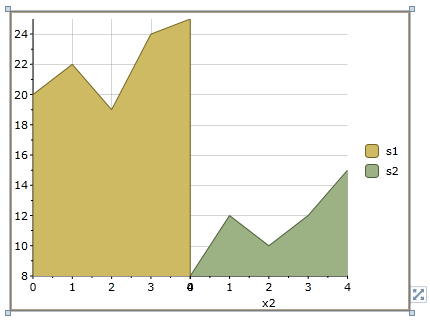You can even stack in both directions at the same time by adding additional X and Y axes.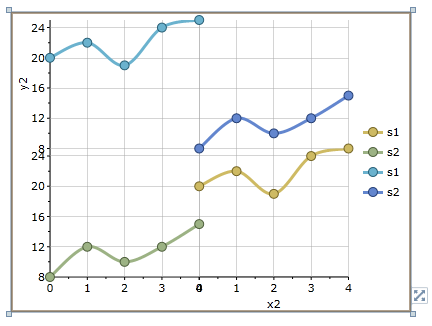If you’d like to apply a different style to each plot area then you can define the areas in XAML and work with them that way. Notice above that it's not very clear where each plot begins and ends. Each PlotArea has several properties you can set for styling including Background, StrokeThickness and Stroke. You determine which plot area is which based upon the Row and Column properties. Here’s a snippet of XAML showing the complete ChartView.

``````
<c1:C1Chart.View>
<c1:ChartView>
<!-- Aux axis -->
<c1:Axis x:Name="y2" Title="y2" AxisType="Y" PlotAreaIndex="1" />
<c1:ChartView.PlotAreas>
<c1:PlotArea Background="LightBlue" Stroke="Blue" Row="1" />
<c1:PlotArea Background="LightGreen" Stroke="Green" Row="0" />
</c1:ChartView.PlotAreas>
</c1:ChartView>
</c1:C1Chart.View>

``````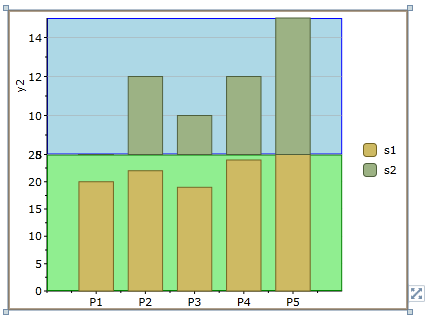My choice of color may not be perfect, but you get the idea. Plot areas can also be created in code using the same idea of creating an axis and settings its PlotAreaIndex property. For example:

``````
// create aux axis for new plot area
Axis y2 = new Axis();
y2.AxisType = AxisType.Y;
y2.Title = "y2";
y2.PlotAreaIndex = 1;

``````

That covers most of the basics for working with multiple plot areas. It’s very easy to pre-set a C1Chart with any number of plot areas, axes and data series. But what if you could allow the end-user to add and remove plot areas on the fly? Then they could drag-and-drop data series among the plots to create their own display. See the next heading.

# Adding and Removing Plot Areas at Run-time

So far we’ve seen how plot areas work. Now let’s take it to the next level by enabling run-time plot area manipulation! I’m not going to post the code but rather just give you the completed sample. The sample you can download below has the following features:

• Dynamically drag-and-drop data series from a TreeView onto the chart
• Add/Remove plot areas above and below each existing area
• Drag-and-drop data series between the plot areas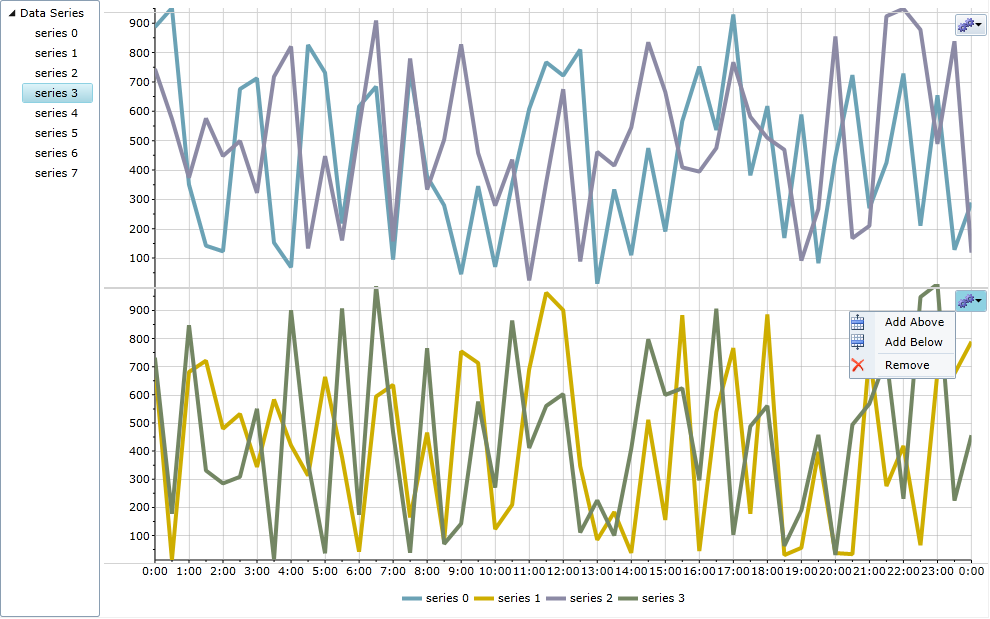To accomplish the interaction we will need to add support for dragging and dropping. For this we can use the C1DragDropManager class. C1DragDropManager makes it easy to register drag and drop targets. In this sample we are dragging items from a C1TreeView, but you could easily configure this part to be anything you want. We will also need to create a mini-toolbar that displays on the perimeter of each plot with the commands for adding and removing plot areas. The toolbar is done using C1Toolbar. In the sample we create a custom "ChartPane" UserControl which enables us to have each plot area as a registered drop target and it displays the toolbar on the right edge for us quite easily. Download the complete sample of your choice below and be sure to also have Studio for Silverlight or Studio for WPF installed. Download Sample - Silverlight 5 Download Sample - WPF 4.0Greg Lutz